﻿ 卷积神经网络的贴片电阻识别应用
«上一篇文章快速检索 高级检索

 智能系统学报2019, Vol. 14Issue (2): 263-272  DOI: 10.11992/tis.2017100050

### 引用本文CHEN Guihui, HE Long, LI Zhongbing, et al. Chip resistance recognition based on convolution neural network[J]. CAAI Transactions on Intelligent Systems, 2019, 14(2): 263-272. DOI: 10.11992/tis.201710005.### 文章历史

Chip resistance recognition based on convolution neural network
CHEN Guihui , HE Long, LI Zhongbing , KANG Yuxin , JIANG Xiaoyu
School of Electrical Information, Southwest Petroleum University, Chengdu 610500, China
Abstract: Chip resistors are widely used in intelligent electronic devices because of their unique properties such as small size and stable performance. The chip resistors produced by the factory must be identified for defects in both front and back faces, polarity, and type in order to guarantee the quality. However, such identification largely relies on the eye detection, which is inefficient, prone to error, and costly. In this paper, considering the limitation of the traditional image recognition methods and the great achievements of convolutional neural network (CNN) in image recognition in recent years, three CNN models, AlexNet model, GoogLeNet model, and ResNet model, with appropriate depth and training parameters of about 4M (million) are designed to overcome the demerits of low speed that results in the inability to meet the real-time requirement. These models overcome the low accuracy problem of generalization recognition associated with the prevailing CNN models, which is caused by many trainable parameters and many layers of model. Experiments show that the recognition accuracy of these three models exceeds 90%. The highest recognition accuracy rate is 95%, and the recognition speed is 0.203 s/piece (256 × 256 pixels, CORE I5). Therefore, these three CNN models can be adopted in practice and have a strong feasibility and replicability; thus, they have a great potential to improve the production efficiency and product quality for chip resistors.
Key words: Chip resistance recognition    convolution neural network    AlexNet model    GoogLeNet model    ResNet model

1 贴片电阻识别任务分析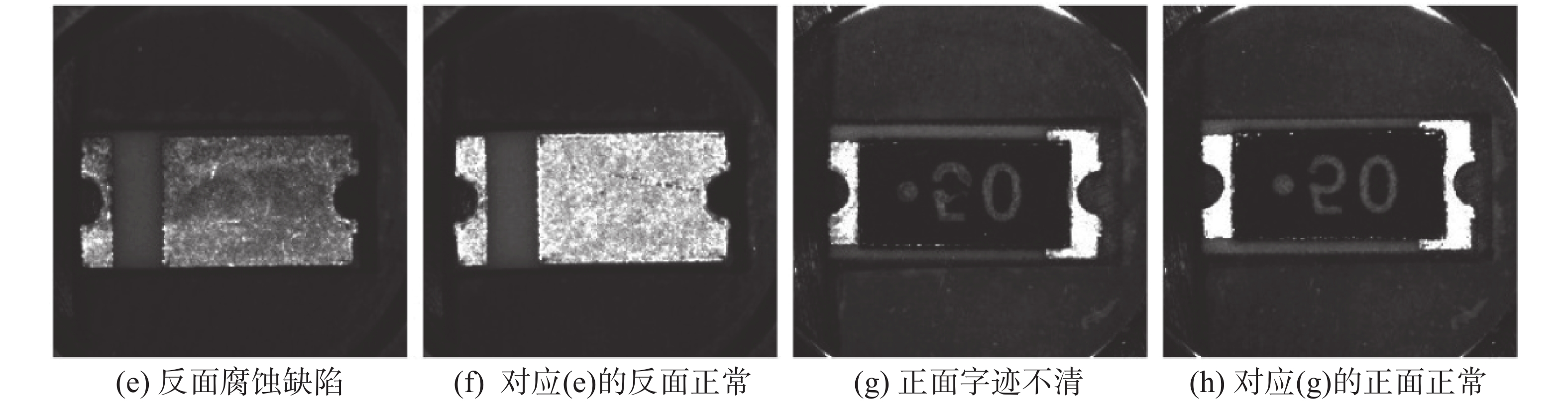Download: 图 1 一种典型电阻型号需识别种类数 Fig. 1 A typical resistance type is required to identify the number of categories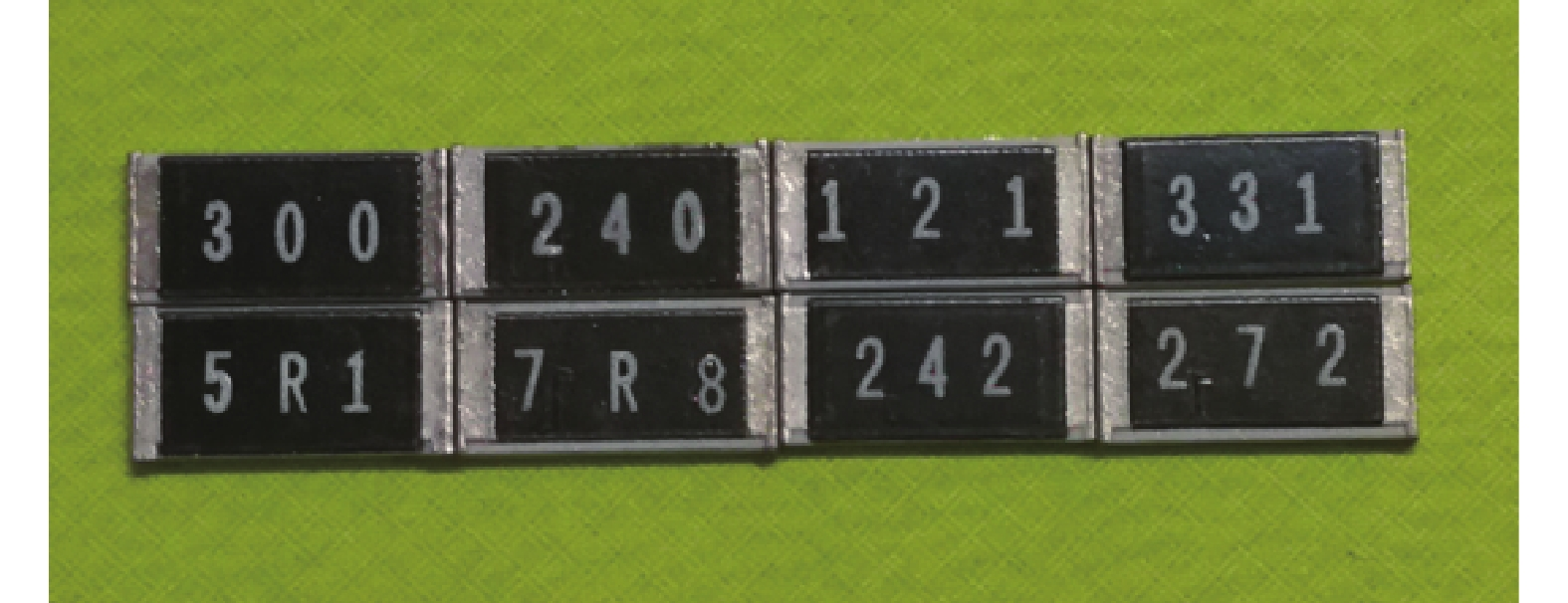Download: 图 2 需要识别的不同型号的贴片电阻示意 Fig. 2 Different types of chip resistors that need to be identified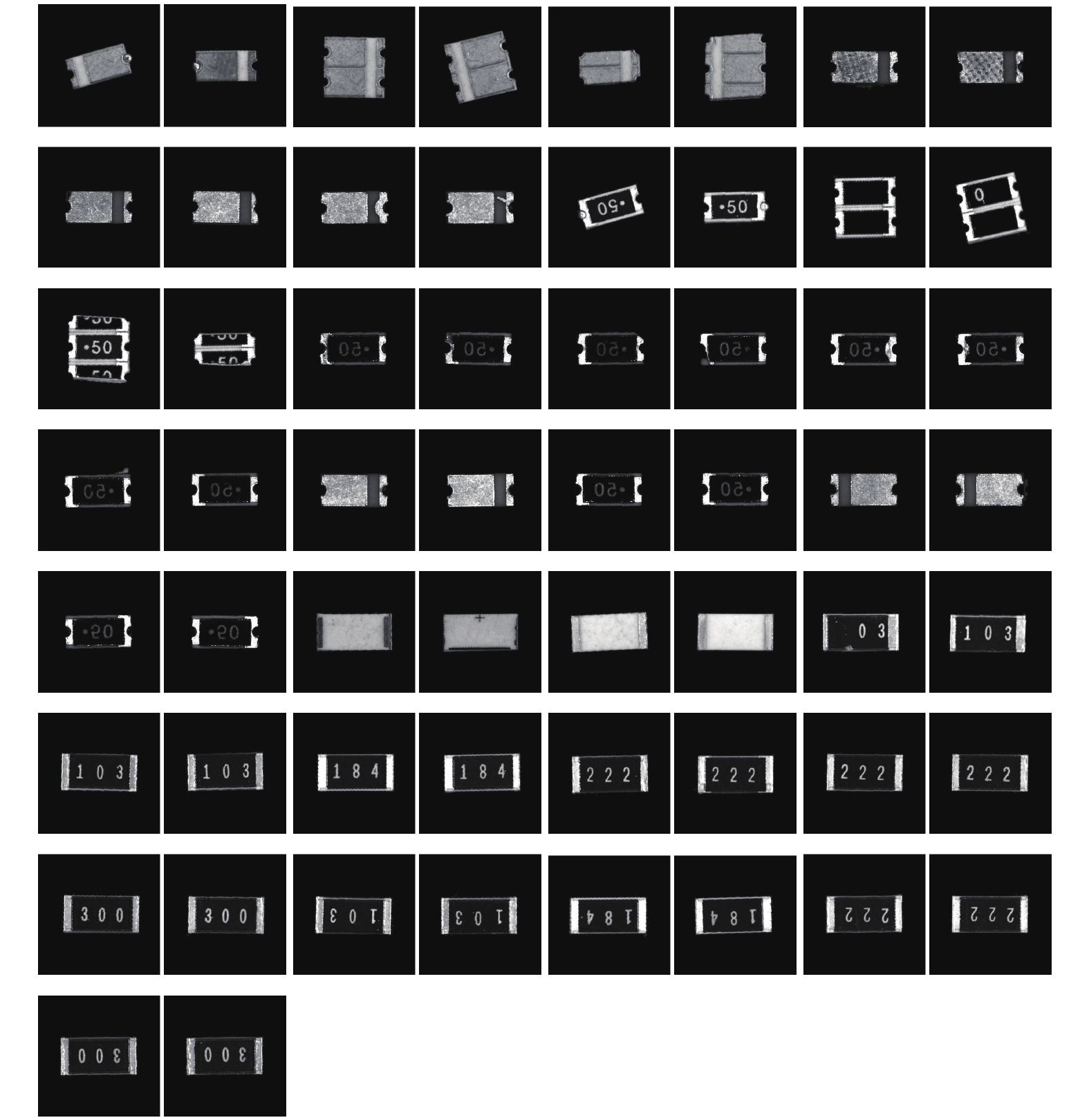Download: 图 3 预处理后的29种贴片电阻图片样例 Fig. 3 Samples of 29 kinds of chip resistors after preprocessing表 1 实验详细数据表 Tab.1 Experimental detailed data sheet
2 卷积神经网络相关数学理论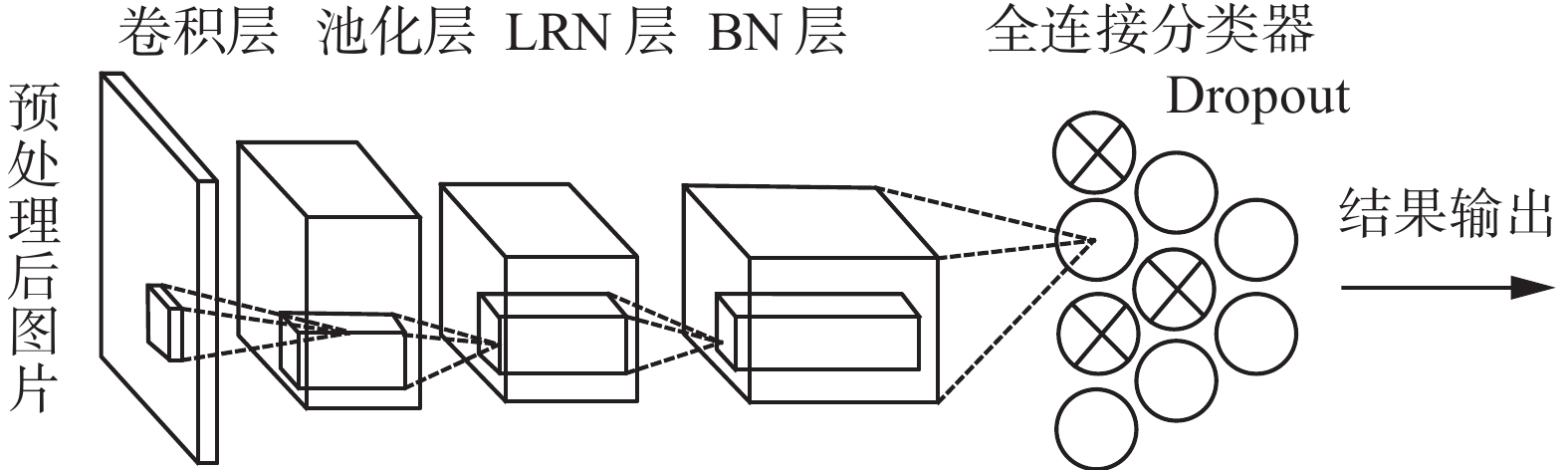Download: 图 4 常见卷积模型图 Fig. 4 Common convolution model diagram
2.1 卷积层模型

 $\begin{array}{c} O(m,n){\rm{ = }}I(m,n)*H(m,n){\rm{ = }} \\ \displaystyle\sum\limits_{m = 0}^{M - 1} {\sum\limits_{n = 0}^{N - 1} {I(i,j)H(m - i,n - j)} }{\rm{ = }} \\ \displaystyle\sum\limits_{i = 0}^{I - 1} {\sum\limits_{j = 0}^{J - 1} {I(m - i,n - j)H(i,j)} } \\ \end{array}$ (1)

 $\begin{gathered} {O^l}\left( {m,n} \right) = {I^k}\left( {m,n} \right) \otimes {H^{kl}}\left( {m,n} \right) = \hfill \\ \sum\limits_{k = 0}^{K - 1} {\sum\limits_{i = 0}^{I - 1} {\sum\limits_{j = 0}^{J - 1} {{I^k}\left( {m + i,n + j} \right){H^{kl}}\left( {i,j} \right)} } } \hfill \\ \end{gathered}$ (2)

2.2 ReLU非线性函数

 $f\left( x \right) = \left\{ {\begin{array}{*{20}{c}} x,&{x > 0} \\ 0,&{x \leqslant 0} \end{array}} \right.$ (3)

2.3 Dropout(随机丢弃)层

Dropout层是为了增加神经网络的稀疏性而特别设计的，其背后的理论依据是为了模仿大脑的稀疏特性。人脑的神经元大多处于抑制状态，只有不到10%的神经元处于活动状态，因此，人脑是一个典型的稀疏结构，每次训练网络通过选择性的丢弃某些神经元，使其不参与前向传播和反向传播过程，一方面可以增加网络的稀疏性，另一方面提供了正则化一大类模型的方法。

2.4 Pooling(池化)层

Pooling层是为了快速降低特征图维度而设计的，从而减轻计算的负担。Pooling层通过汇总上一层特征图相邻神经元的输出，输出一个维度被降低的特征图。通过Pooling层的操作，模型计算量被减少，网络过拟合问题得到一定的缓解，网络整体性能也有所提升。常见的汇总操作有MAX和AVE，MAX操作为局部相邻神经元的最大值作为输出，AVE操作为局部相邻神经元的平均值作为输出，与图像均值滤波类似。

2.5 LRN(local response normalization)层

LRN层为局部响应值归一化层，LRN层跟随在Pooling层之后，通过对局部神经元的活动创建竞争机制，使得其中响应比较大的值变得相对更大，并抑制其他反馈较小的神经元，保留关键特征，抑制非重要特征。LRN层具体归一化公式如式(4)所示：

 $b_{x,y}^i = a_{x,y}^i/{\left( {k + \alpha \sum\limits_{j = \max (0,i - n/2)}^{\min (N - 1,i + n/2)} {{{\left( {a_{x,y}^j} \right)}^2}} } \right)^\beta }$ (4)

2.6 BatchNorm层

BatchNorm层为步长归一化层，卷积神经网络在读入训练数据和测试数据时，并不是每次只读取一张图片，而是一次读取一个Batch(步长)值的图片数据。这样就带来一个问题，深度神经网络每一层的输入分布在训练的时候会发生改变，导致卷积神经网络难以学习到数据中蕴藏的本质，这使得要训练这样的网络变得困难复杂，而这样的问题可以通过归一化每一层的输入来解决。BN的本质作用原理是强行将每一层输入分布的变化进行矫正，使得每一层输入在进行卷积之前保持零均值和单位方差。使用BN层的深度网络结构，可以使用更高的学习率进行训练，而且也不用过多的关心训练权值初始化问题。实践证明加入BN层的深度网络不仅训练收敛速度极大提高，而且识别准确率也得到改善。

3 卷积模型架构设计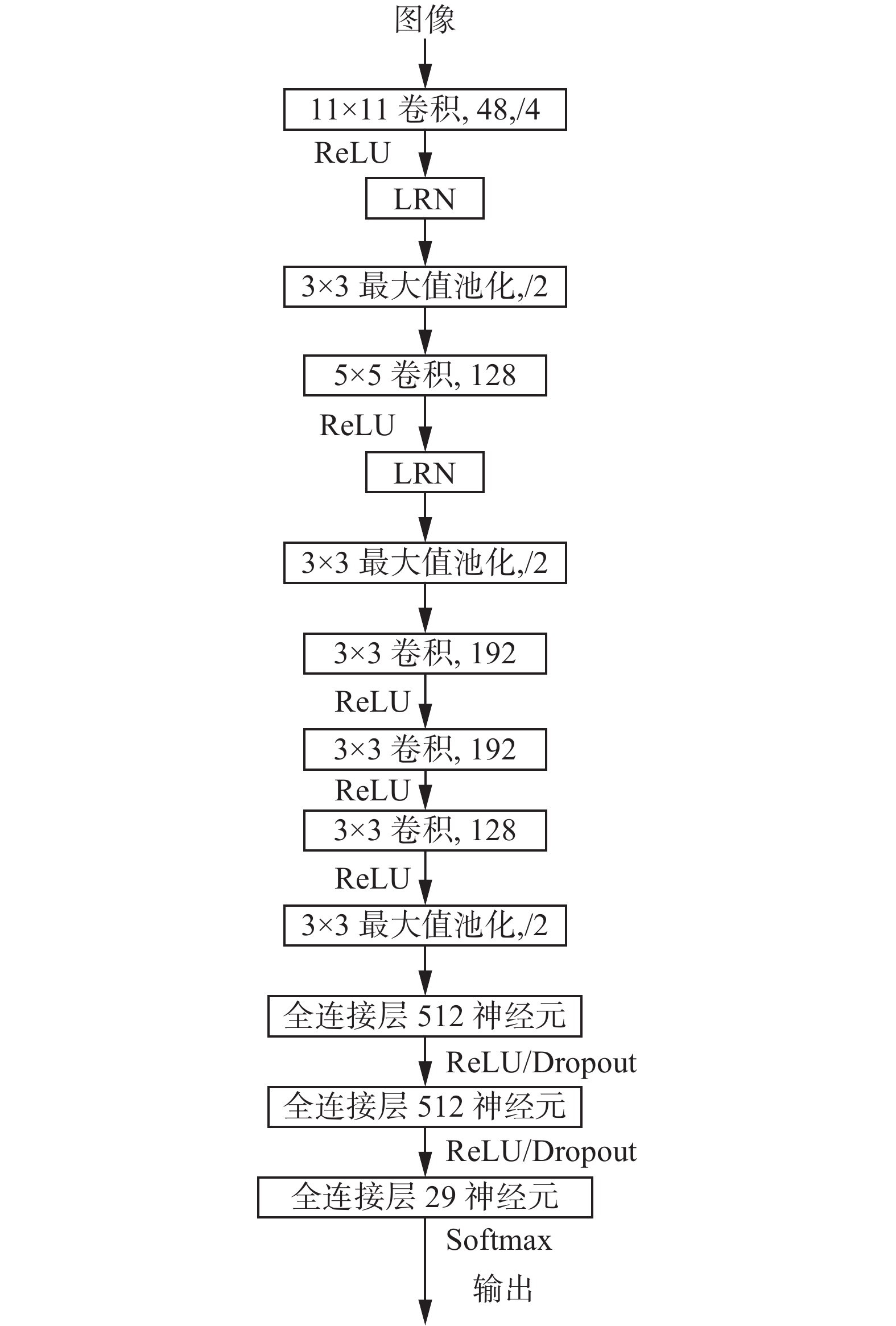Download: 图 5 识别贴片电阻的8层经典卷积网络结构，可训练参数为4.07×106 Fig. 5 The 8-layer classical convolution network structure of identify the chip resistors with a training parameter of 4.07×106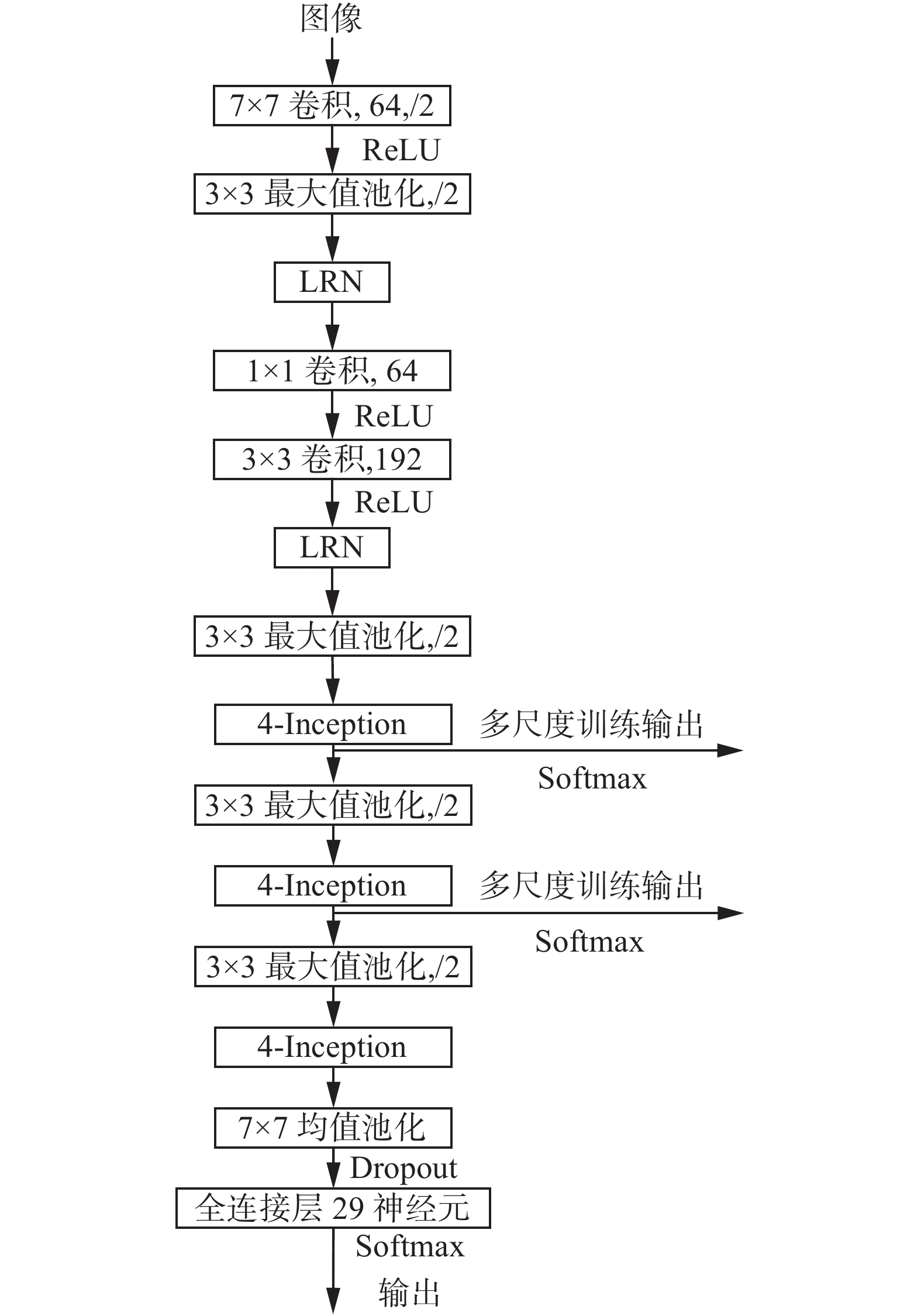Download: 图 6 识别贴片电阻的16层深度4-Inception模型图，可训练参数为3.8×106 Fig. 6 The 16-layer depth 4-Inception model of identify the chip resistors with a training parameter of 3.8×106表 2 4-Inception详细参数设置 Tab.2 4-Inception detailed parameter settings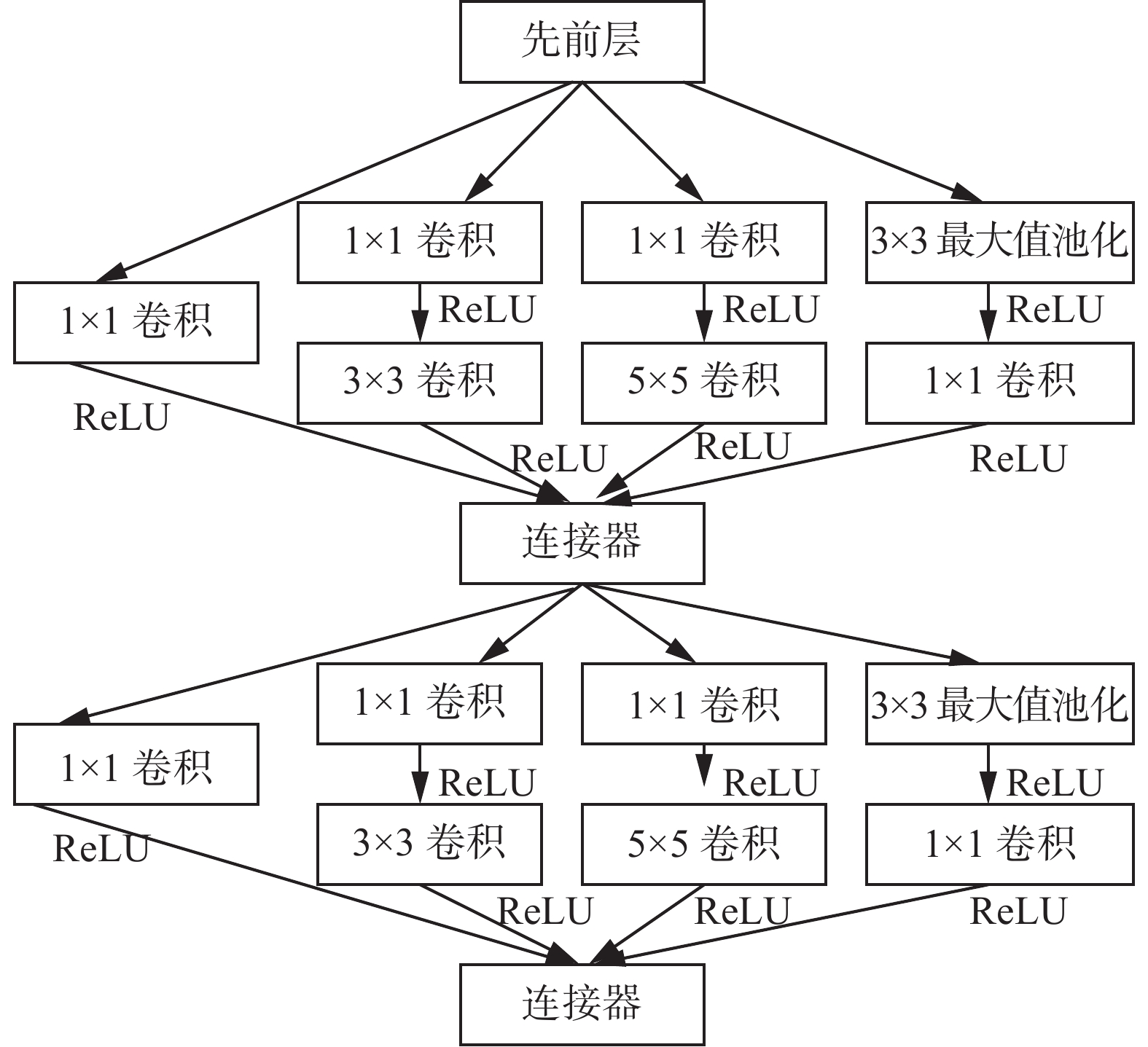Download: 图 7 谷歌Inception卷积结构 (4-Inception) Fig. 7 Google Inception convolution structure (4-Inception)

 $\frac{{\partial \left( {f(x) + x} \right)}}{{\partial x}} = \frac{{\partial f(x)}}{{\partial x}} + 1$ (5)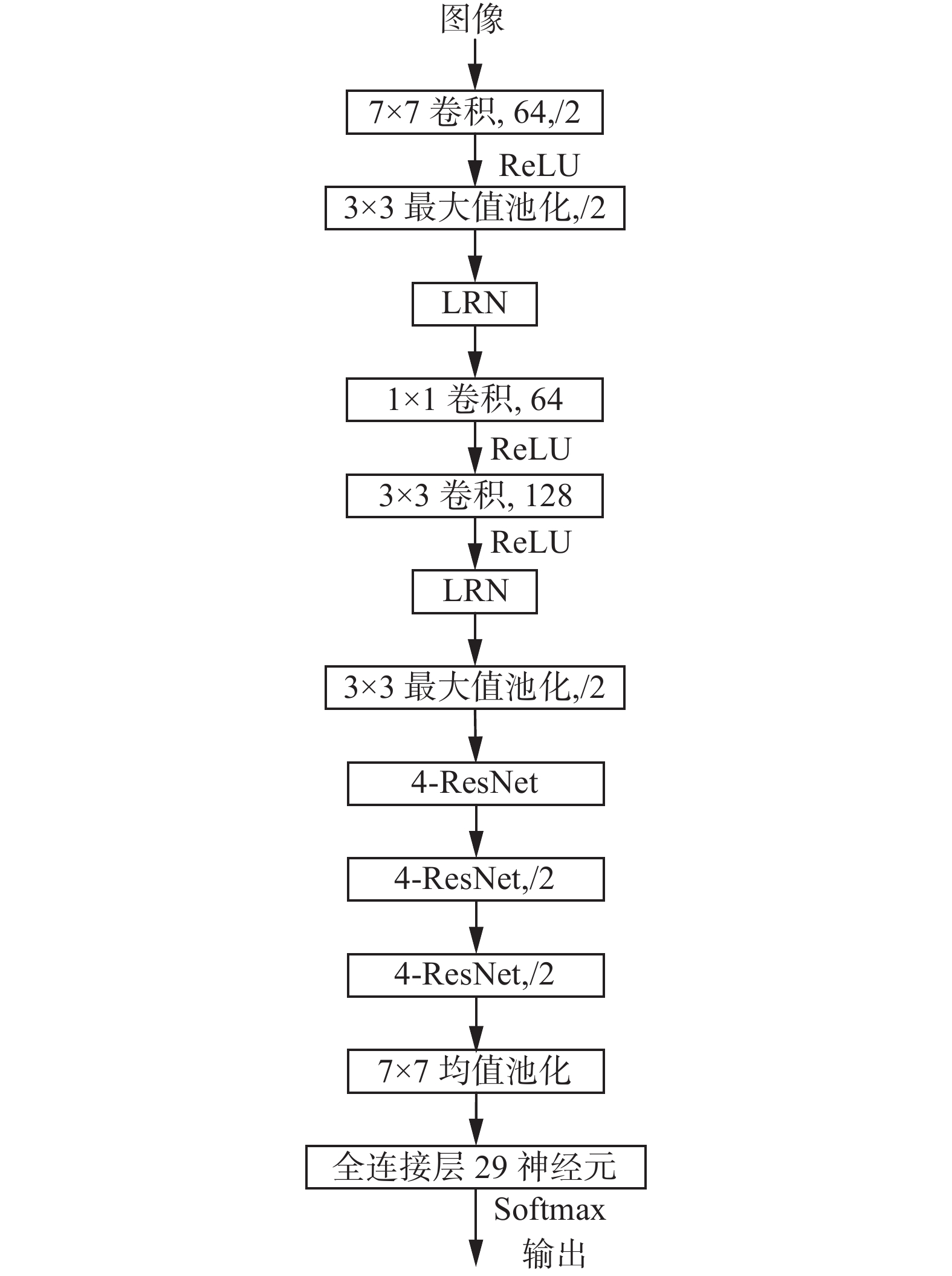Download: 图 8 识别贴片电阻的16层深度4-ResNet模型，可训练参数为4.0×106 Fig. 8 The 16-layer depth 4-ResNet model of identify the chip resistors with a training parameter of 4.0×106

3种模型的输出神经元均被设置成29，这是由实验数据集包含29个类别所导致的，训练时均采用随机梯度下降法进行训练，选择Softmax回归模型对网络进行优化，权值更新公式为

 $\begin{array}{c} {v_{i + 1}} = 0.9 \times {v_i} - 0.000\;5 \times \varepsilon \times {w_i} - \varepsilon \times {\left\langle {{{\left. {\displaystyle\frac{{\partial L}}{{\partial w}}} \right|}_{{w_i}}}} \right\rangle _{{D_i}}} \hfill \\ {w_{i + 1}} = {w_i} + {v_{i + 1}} \hfill \end{array}$ (6)

4 实验及讨论 4.1 贴片电阻识别指标定义

 $N = \sum\limits_{i = 1}^m {{n_i}}$ (7)

 $T = \sum\limits_{i = 1}^m {{t_i}}$ (8)

 $E = \sum\limits_{i = 1}^m {{e_i}}$ (9)

 ${\rm{acc}}\_{\rm{all}} = \left( {1 - \frac{E}{T}} \right)\times100{\text{%}}$ (10)

 ${\rm{err}}\_{\rm{all}} = {\frac{e_i}{T}}\times 100{\text{%}}$ (11)

 ${\rm{acc}}\_i = \left( {1 - \frac{{{e_i}}}{{{t_i}}}} \right)\times 100{\text{%}}$ (12)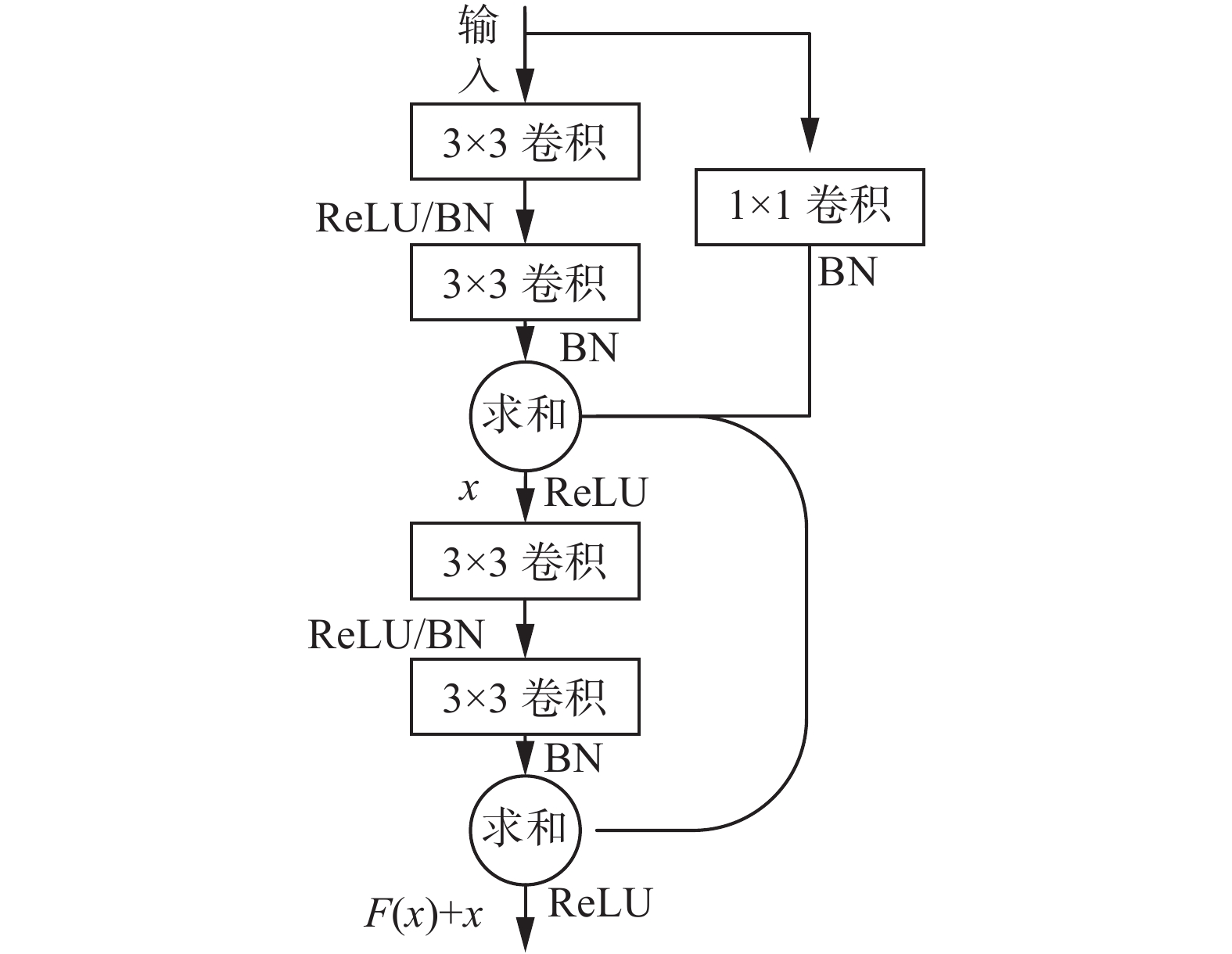Download: 图 9 残差网络结构，取名4-ResNet Fig. 9 Residual network structure, named 4-ResNet
4.2 实验过程

4.3 实验结果分析

3种模型的训练结果分别如图10~11所示，可以看出，由于贴片电阻位置的随机性，识别准确率最高的为16层4-Inception网络，识别准确率达到95%，这说明层数更深的4-Inception网络更加擅长处理复杂情况下的图像识别，特征解耦更好，更适合贴片电阻识别分类任务。残差网络是收敛最快的网络结构，但其测试损失波动非常大并在迭代2 500次后趋于平稳，继续训练则是过拟合训练，因此实验时训练迭代次数未到7 200次则将其停止。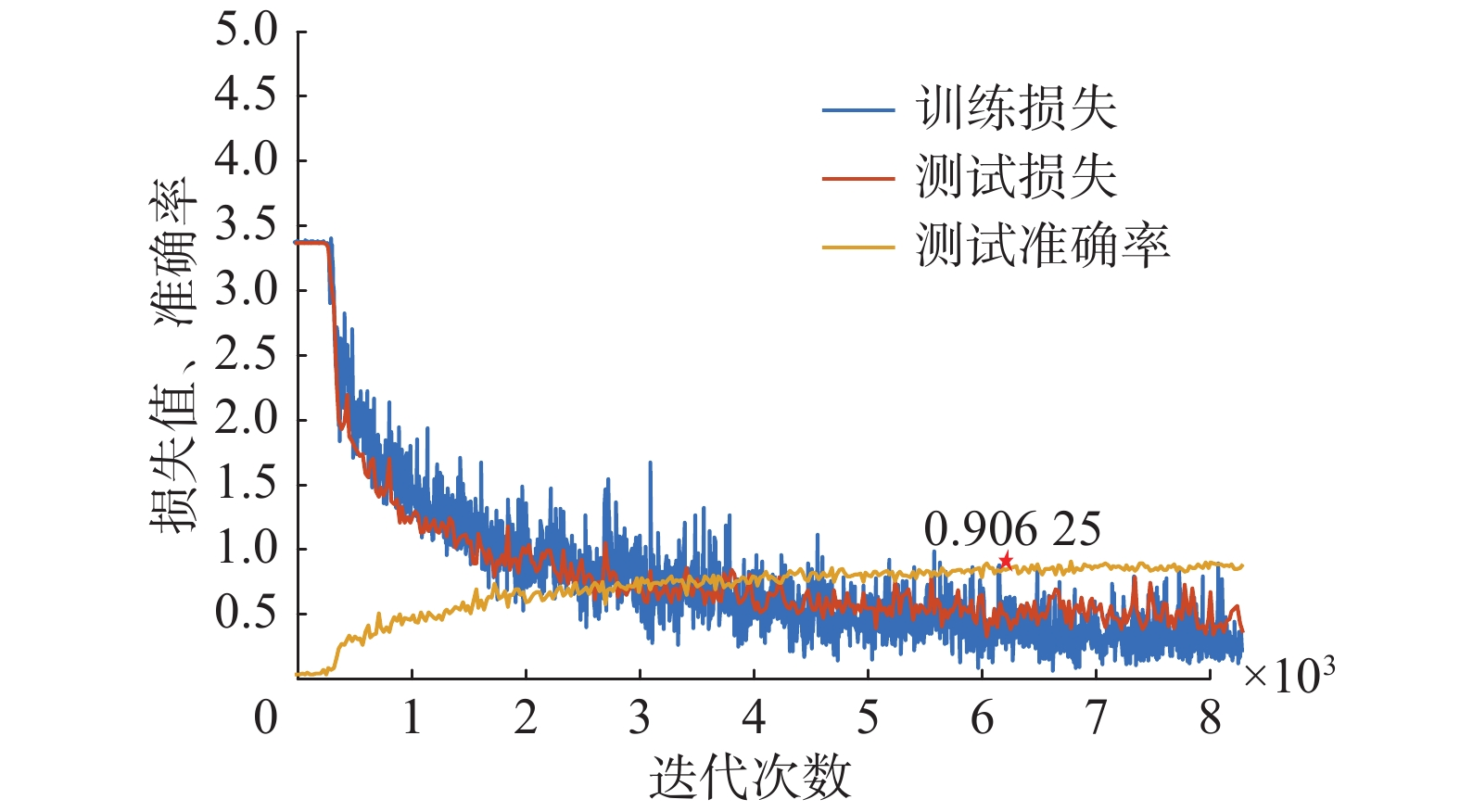Download: 图 10 贴片电阻位置随机8层深度卷积模型训练结果 Fig. 10 Chip resistor position random 8-layer depth convolution model training results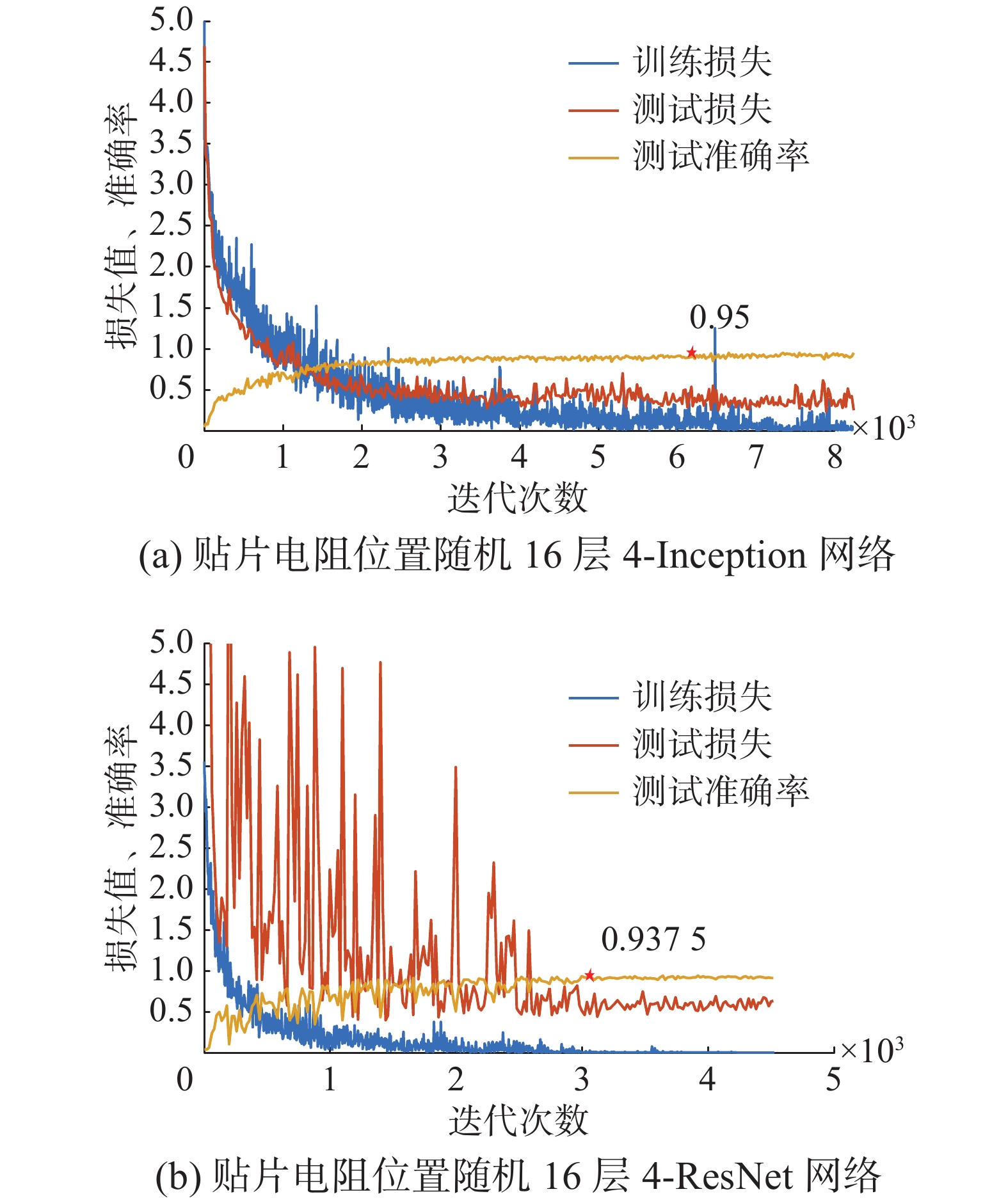Download: 图 11 网络训练结果 Fig. 11 Network training results

3种模型性能详细对比如表3所示，识别时间最快的是8层深度卷积网络，低至0.203 秒/张(256×256像素/COREI5)，识别准确率最高的是16层4-Inception网络，识别准确率达95%，残差网络性能折中。表 3 3种模型识别时间及准确率对比 Tab.3 Comparison of 3 model identification times and accuracy

3种模型的泛化识别错误率分布如图12~13所示，可以看出，标签1~13主要属于缺陷类别识别，是识别错误率的主要来源。标签14~29主要识别正反面、方向和种类，3种模型均取得了非常理想的识别效果。图13(a)中的16层4-Inception模型，其仅在缺陷识别上存在一定的错误率，在种类、正反面和方向识别上取得了100%的识别准确率。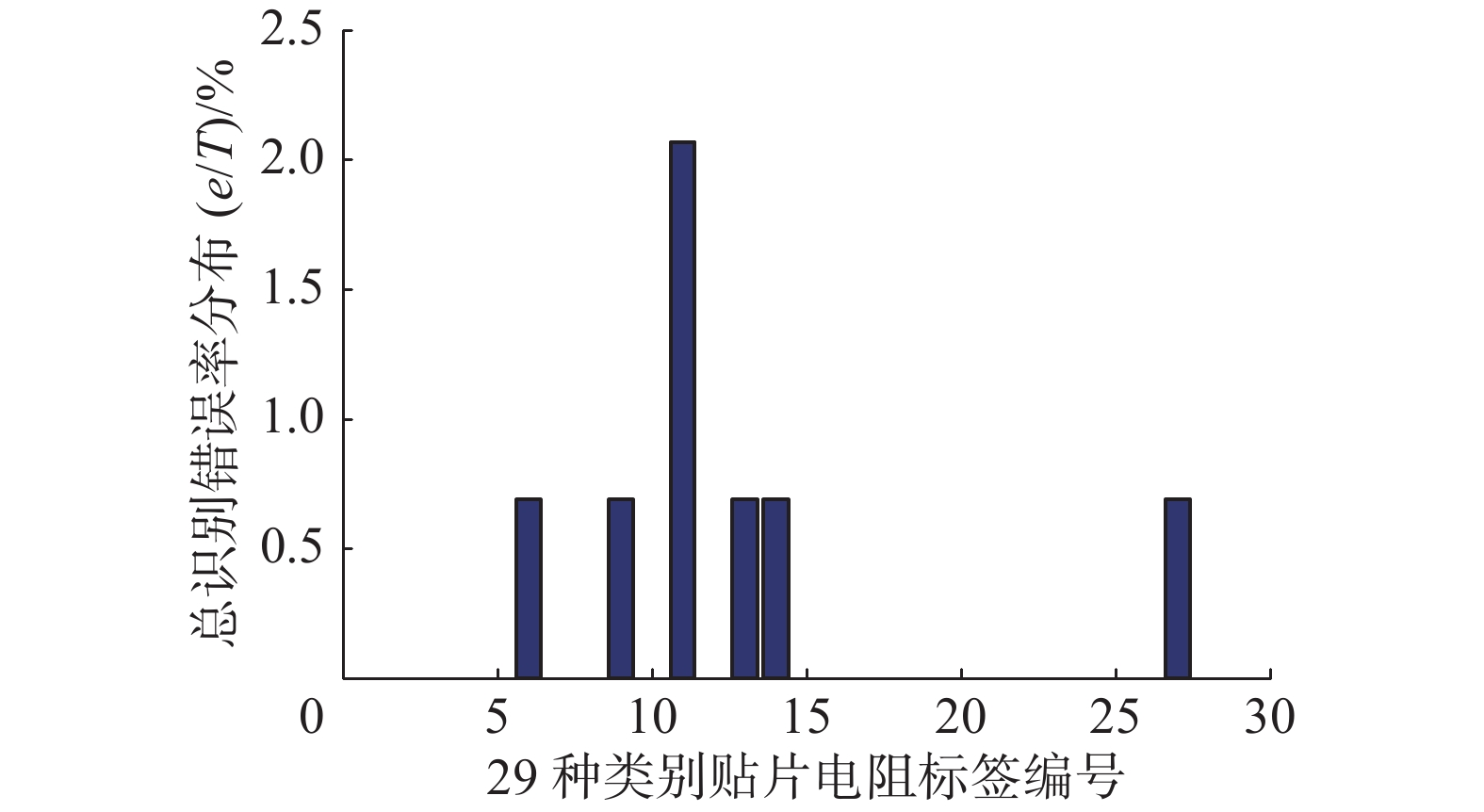Download: 图 12 8层深度卷积网络总识别错误率分布 Fig. 12 Total error rate distribution of 8-layer deep convolutional networks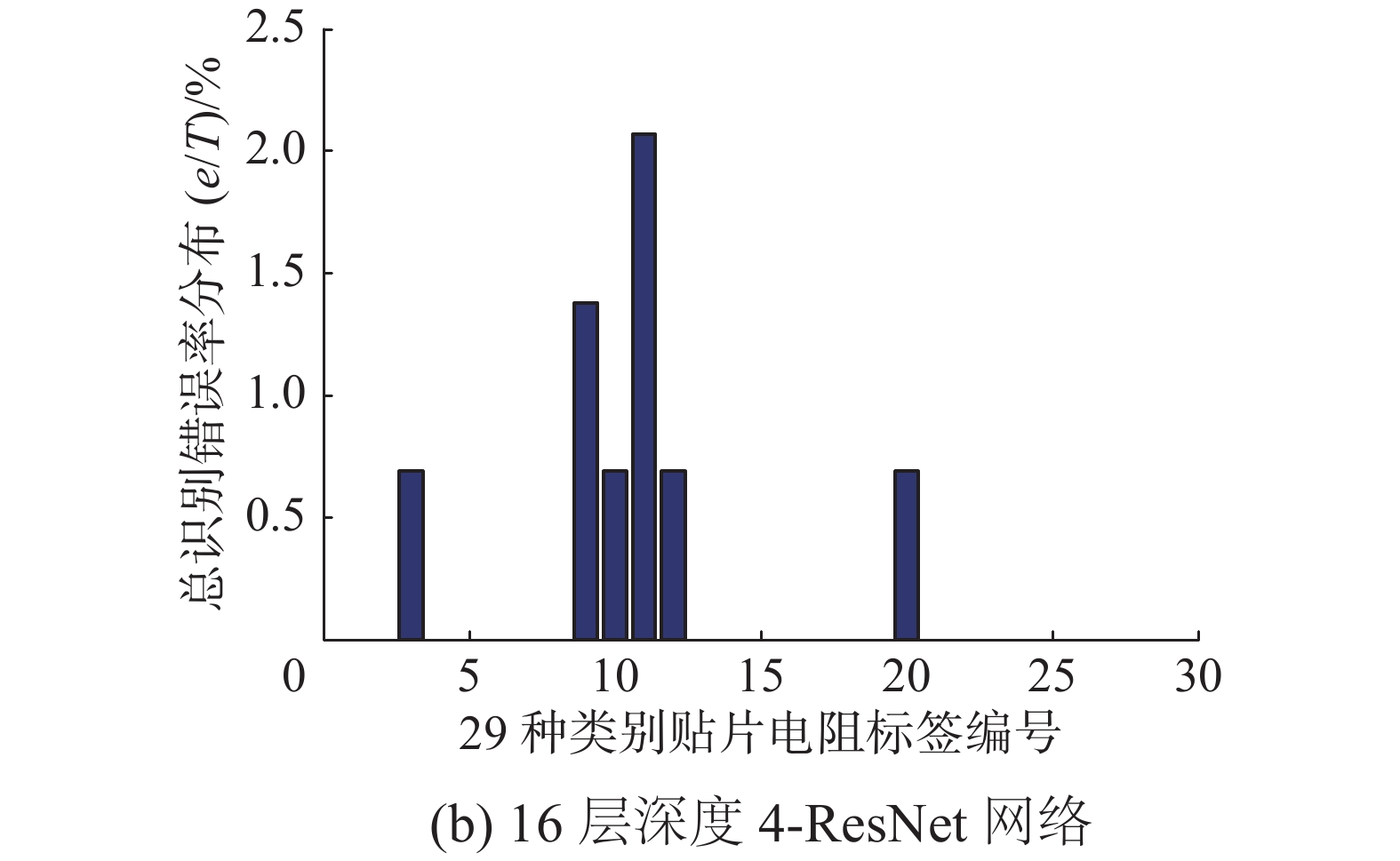Download: 图 13 网络总识别错误分布 Fig. 13 Networks total error rate distribution

5 结束语

  GIUNCHIGLIA F, YATSKEVICH M. Element level semantic matching. Technical Report DIT-04-035[R]. Trento, Italy: Information Engineering and Computer Science, 2004. (0)  OMACHI M, OMACHI S. Fast two-dimensional template matching with fixed aspect ratio based on polynomial approximation[C]//Proceedings of the 9th International Conference on Signal Processing. Beijing, China, 2008: 757–760. (0)  JOLLIFFE I T. Principal component analysis[M]. New York, USA: Springer-Verlag, 1986. (0)  ZHANG Xingfu, REN Xiangmin. Two dimensional principal component analysis based independent component analysis for face recognition[C]//Proceedings of 2011 International Conference on Multimedia Technology. Hangzhou, China, 2011: 934–936. (0)  PELLEGRINO F A, VANZELLA W, TORRE V. Edge detection revisited[J]. IEEE transactions on systems, man, and cybernetics, part b (cybernetics), 2004, 34(3): 1500-1518. DOI:10.1109/TSMCB.2004.824147 (0)  DUCOTTET C, FOURNEL T, BARAT C. Scale-adaptive detection and local characterization of edges based on wavelet transform[J]. Signal processing, 2004, 84(11): 2115-2137. DOI:10.1016/j.sigpro.2004.07.003 (0)  SMITH S M, BRADY J M. SUSAN-a new approach to low level image processing[J]. International journal of computer vision, 1997, 23(1): 45-78. DOI:10.1023/A:1007963824710 (0)  CORTES C, VAPNIK V. Support-vector networks[J]. Machine learning, 1995, 20(3): 273-297. (0)  GUO H, GELFAND S B. Classification trees with neural network feature extraction[J]. IEEE transactions on neural networks, 1992, 3(6): 923-33. DOI:10.1109/72.165594 (0)  GOLDSZMIDT M. Bayesian network classifiers[J]. Machine learning, 2011, 29(2/3): 598-605. (0)  焦李成, 杨淑媛, 刘芳, 等. 神经网络七十年: 回顾与展望[J]. 计算机学报, 2016, 39(8): 1697-1717. JIAO Licheng, YANG Shuyuan, LIU Fang, et al. Seventy years beyond neural networks: retrospect and prospect[J]. Chinese journal of computers, 2016, 39(8): 1697-1717. (0)  HINTON G E, SALAKHUTDINOV R R. Reducing the dimensionality of data with neural networks[J]. Science, 2006, 313(5786): 504-507. DOI:10.1126/science.1127647 (0)  ERHAN D, MANZAGOL P A, BENGIO Y, et al. The difficulty of training deep architectures and the effect of unsupervised pre-training[C]//Appearing in Proceedings of the 12th International Conference on Artificial Intelligence and Statistics. Florida, USA, 2009: 153–160. (0)  LECUN Y, BENGIO Y. Convolutional networks for images, speech, and time series[M]//ARBIB M A. The Handbook of Brain Theory and Neural Networks. Cambridge, MA, USA: MIT Press, 1998. (0)  KRIZHEVSKY A, SUTSKEVER I, HINTON G E. Imagenet classification with deep convolutional neural networks[C]//International Conference on Neural Information Processing Systems. Montreal, Canada, 2012: 1097–1105. (0)  SZEGEDY C, LIU Wei, JIA Yangqing, et al. Going deeper with convolutions[C]//Proceedings of 2015 IEEE Conference on Computer Vision and Pattern Recognition. Boston, MA, USA, 2014: 1–9. (0)  SZEGEDY C, IOFFE S, VANHOUCKE V, et al. Inception-v4, inception-resnet and the impact of residual connections on learning[J]. arXiv: 1602.07261, 2016. (0)  HE Kaiming, ZHANG Xiangyu, REN Shaoqing, et al. Deep residual learning for image recognition[C]//Proceedings of 2016 IEEE Conference on Computer Vision and Pattern Recognition. Las Vegas, NV, USA, 2016: 770–778. (0)  IOFFE S, SZEGEDY C. Batch normalization: accelerating deep network training by reducing internal covariate shift[C]//Proceedings of the 32nd International Conference on Machine Learning. Lille, France, 2015: 448–456. (0)  GLOROT X, BORDES A, BENGIO Y. Deep sparse rectifier neural networks[C]//Proceedings of the 14th International Conference on Artificial Intelligence and Statistics. Fort Lauderdale, FL, USA, 2011: 315–323. (0)